# How to Count Rows With Criteria Using SUMPRODUCT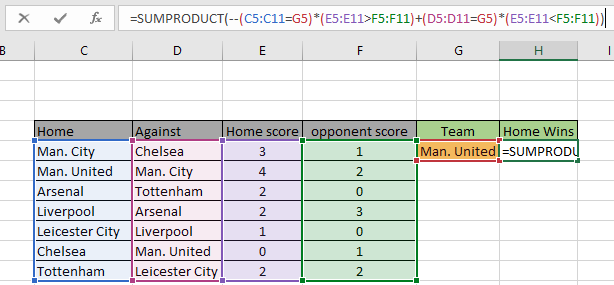In this article, we will learn how to count rows with criteria SUMPRODUCT in Excel.

Scenario:
In simple words, while working data, sometimes we need to count the cells where 2 ranges meet criteria. The conditional count can be done using COUNTIF function but this is not the only function that can do so. SUMPRODUCT function is a versatile function that can be used to count with criteria, sometimes even better than countif function.

How to solve the problem?
For this article we will be required to use the SUMPRODUCT function. Now we will make a formula out of these functions. Here we are given two ranges data and we need to count the values where the formula satisfies criteria.

Generic formula:

= SUMPRODUCT ( -- ( range1 operator range2 ) )

range1 : 1st range
range2 : 2st range
operator : criteria, condition given as operator between the 2 ranges

Example:
All of these might be confusing to understand. So, let's test this formula via running it on the example shown below.

Here we have the record for the last 7 big matches of Premier League Fan Clubs.

We have the record for the last 7 matches having Home team, Against team, Home scored & opponent scored goals.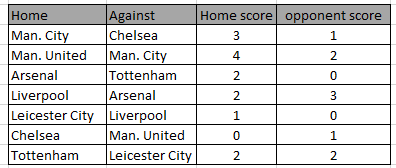Firstly, we need to find the count of games won at home by any team.

Now we will use the following formula to get the count of games who won at home.
Use the Formula:

= SUMPRODUCT ( -- ( E5:E11 > F5:F11 ) )

E5:E11 : 1st range, home scored goals
F5:F11 : 2st range, opponent scored goals
> : criteria, condition given as greater than the operator

Explanation:

• The condition here which needs to be satisfied is the home scored goals must be greater than opponent scored goals.
• E5:E11 ( range1 ) must be greater than F5:F11 ( range2 ).
• -- operator used to convert TRUE value to 1 & False value to 0.
• SUMPRODUCT function gets the sum of 1s in the returned array, which will be the count of required wins.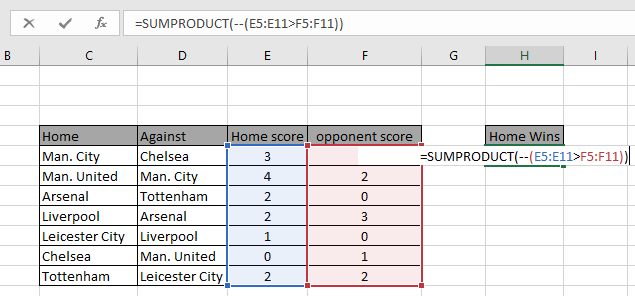Here the range is given as cell reference. Press Enter to get the count.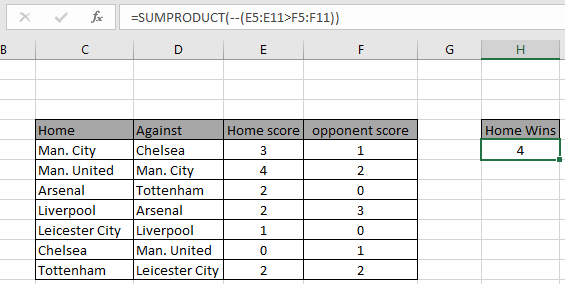As you can see the total matches where the home team had the advantage and won are 4 matches.

The above example was easy. So to make it interesting we will count how many matches a team won in the whole data.Firstly, we need to find the count of games won at home by any team.

Now we will use the following formula to get the count of games, Man. United won.
Use the Formula:

= SUMPRODUCT ( -- ( C5:C11 = G5 ) * ( E5:E11 > F5:F11 ) + ( D5:D11 = G5 ) * ( E5:E11 < F5:F11 ) )

Explanation:

• (C5:C11 = G5 ) * ( E5:E11 > F5:F11 ) checks the home team as Man. United and scored more goals than the opponent team.
• ( D5:D11 = G5 ) * ( E5:E11 < F5:F11 ) checks the against team as Man. United and scored more goals than the home team.
• SUMPRODUCT function gets the sum of 1s in the returned array, which will be the count of required wins by Man. United.Here the range is given as cell reference. Press Enter to get the count.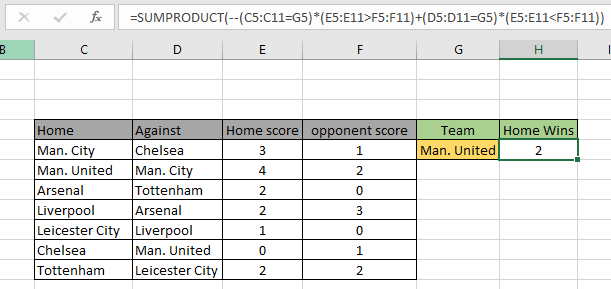As you can see the total matches where the Man. The United team won 2 matches.

Here are some observational notes shown below.

Notes:

1. The formula only works with numbers.
2. The formula only works when there are no duplicates on the lookup table
3. The SUMPRODUCT function considers non- numeric values as 0s.
4. The SUMPRODUCT function considers logic value TRUE as 1 and False as 0.
5. The argument array must be of the same length as the function

Hope this article about how to Return Count if with SUMPRODUCT in Excel is explanatory. Find more articles on SUMPRODUCT functions here. Please share your query below in the comment box. We will assist you.

Related Articles

How to use the SUMPRODUCT function in Excel: Returns the SUM after multiplication of values in multiple arrays in excel.

COUNTIFS with Dynamic Criteria Range : Count cells dependent on other cell values in Excel.

COUNTIFS Two Criteria Matches: Count cells matching two different criteria on the list in excel.

COUNTIFS With OR For Multiple Criteria : Count cells that have multiple criteria that match using the OR function.

The COUNTIFS Function in Excel : Count cells dependent on other cell values.

How to Use Countif in VBA in Microsoft Excel : Count cells using Visual Basic for Applications code.

How to use wildcards in excel : Count cells matching phrases using the wildcards in excel

Popular Articles

50 Excel Shortcuts to Increase Your Productivity

Edit a dropdown list

Absolute reference in Excel

If with conditional formatting

If with wildcards

Vlookup by date

Convert Inches To Feet and Inches in Excel 2016

Join first and last names in excel

Count cells that match either A or B

Terms and Conditions of use

The applications/code on this site are distributed as is and without warranties or liability. In no event shall the owner of the copyrights, or the authors of the applications/code be liable for any loss of profit, any problems or any damage resulting from the use or evaluation of the applications/code.